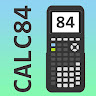# Graphing calculator plus 84 graph emulator free 83

## Information

 Updated 2020-09-01 Rating 0 ★ / 5 version 5.1.0.588 Size 26.52 MB OS Require Android 4.1.x - Jelly Bean Packagename scientific.graphing.calculator.t84.t36.t83 Author's Notes Robust graphing calculator 84, 83, equation solver, algebra, math and fractions

## Description

The full-feature scientific graphing calculator 84 plus provides many useful features for students:
- Graphing calculator 83 ti plus supports graphing, draw graph of many functions such as parametric, polar and functions
- Scientific graphing calculator 84 plus working as well as calculator t1 83 by supporting equation solver, newton root finding, fraction calculation.
- Graphncalc calculator 89 ti plus include integral calculator, symbolic derivative
- Statistics and regression: one variable statistics and two variables statistic, linear regression, quadratic, quartic, ...

This graphing calculator 84 plus ti emulates as an emulator 84 ti plus, students can use as an instruments tools for homework.
The robust equation solver can solve all of equations such as 83 x ^ 4 - 84 x ^ 3 + 89 x = 83
Finally, grafischer taschenrechner 84 ti includes full function documentation and examples.

Graphing calculator plus 84 graph emulator free 83 APK - v5.1.0.588

Updated: 2020-10-19

## What's new

Support zoom commands
Inequality function graph